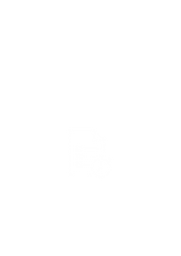YEAR 11Our Objective

The year 11 Advanced Mathematics program at Cosmos aims at helping students understand all the concepts in advanced Maths in detail and score maximum marks in their school assessments and final exam. We achieve this by providing them with the ultimate combination of our interactive lectures, study material, quizzes, worksheets and continuous formative assessments. Students learn the entire year 11 standard Mathematics syllabus as per the latest NSW curriculum in full depth.  Students need to attend two classes every week along with an optional doubt clearing and extra practice class every Sunday. Each class is 2 hours long with a 10-minute break in between. Students are motivated to keep their assessments up to date on google classroom and parents receive report cards every term containing detailed statistics of their children's test scores. Students can ask our teachers to help them personally with their school homework before/after class hours.

Year 11 Maths Tutoring Program

Weekly Course ScheduleResourcesTimetableMathematics Syllabus

Fundamentals of Algebra

Subtopics:

• Arithmetic with pronumerals
• Expanding brackets
• Factoring
• Algebraic fractions
• Solving linear equations
• Solving simultaneous equations
• Completing the square

Indices and Surds

Subtopics:

• Whole numbers, integers and rationals
• Real numbers and approximations
• Surds and their arithmetic
• Further simplification of surds
• Rationalising the denominator

Functions and Graphs

• Functions and function notation
• Functions, relations and graphs
• Review of linear graphs
• Quadratics functions — factoring and the graph
• Completing the square and the graph
• The quadratic formulae and the graph
• Powers, polynomials and circles
• Two graphs that have asymptotes
• Four types of relations

Transformations

Subtopicss:

• Translations of known graphs
• Reflections in the x-axis and y-axis
• Even and odd symmetry
• The absolute value function
• Composite functions

Trigonometry

Subtopics:

• Trigonometry with right-angled triangles
• Problems involving right-angled triangles
• Three-dimensional trigonometry
• Trigonometric functions of a general angle
• Quadrant, sign, and related acute angle
• Given one trigonometric function, find another
• Trigonometric identities
• Trigonometric equations
• The sine rule and the area formula
• The cosine rule
• Problems involving general triangles

The Coordinate Plane

Subtopics:

• Lengths and midpoints of intervals
• Gradients of intervals and lines
• Equations of lines
• Further equations of lines
• Using pronumerals in place of numbers

Exponential and Logarithmic Functions

• Indices
• Fractional indices
• Logarithms
• The laws for logarithms
• Equations involving logarithms and indices
• Exponential and logarithmic graphs
• Applications of these functions

Differentiation

• Tangents and the derivative
• The derivative as a limit x
• rule for differentiating powers of x
• Tangents and normals — dy dx notation
• Differentiating powers with negative indices
• Differentiating powers with fractional indices
• The chain rule
• The product rule
• The quotient rule
• Rates of change
• Continuity
• Differentiability

• The exponential function base
• Transformations of exponential functions
• Differentiation of exponential functions
• Differentiation and the graph
• The logarithmic function base e
• Exponential rates using the base e
• Radian measure of angle size
• Solving trigonometric equations
• Arcs and sectors of circles

Probability

• Probability and sample spaces
• Sample space graphs and tree diagrams
• Sets and Venn diagrams
• Venn diagrams and the addition theorem
• Multi-stage experiments and the product rule
• Probability tree diagrams
• Conditional probabilityWhat

Parents SayOur Students'

Results

Amol Kumar

My child loved the tutoring. He had no interest in science when he started but he now enjoys it. Shariq made the subject really interesting by showing students videos and doing activities in class.

Alvin White

Great place for study. students can come anytime and study in the study rooms and also ask questions to teachers. Sai and his team is extremely helpful.

Yousef Mohammed

Rate 5 stars. Nice campus and learning environment. Students are taught several practical based things apart from regular academics.

Tanishka Reddy

Flexible teaching style. Teachers understood that my child was able to catch things fast and already knew the content so they promoted him to the next class and taught him the full syllabus. My son enjoyed his experience.

Sameer Reddy

Received lot of study material for practice. Online quizzes and tests were pretty helpful. Certainly recommend.

Very good coaching center. Nice staff and location.Natalia J.

Erik S.

Arif B.

Arjun P.

Kritik L.

Test Yourself

Topic

Max Marks

Questions

Exponential and Log Functions

Differentiation

Surds and Indices

Trigonometry

50

50

50

50

10

10

10

10

Let's do this!